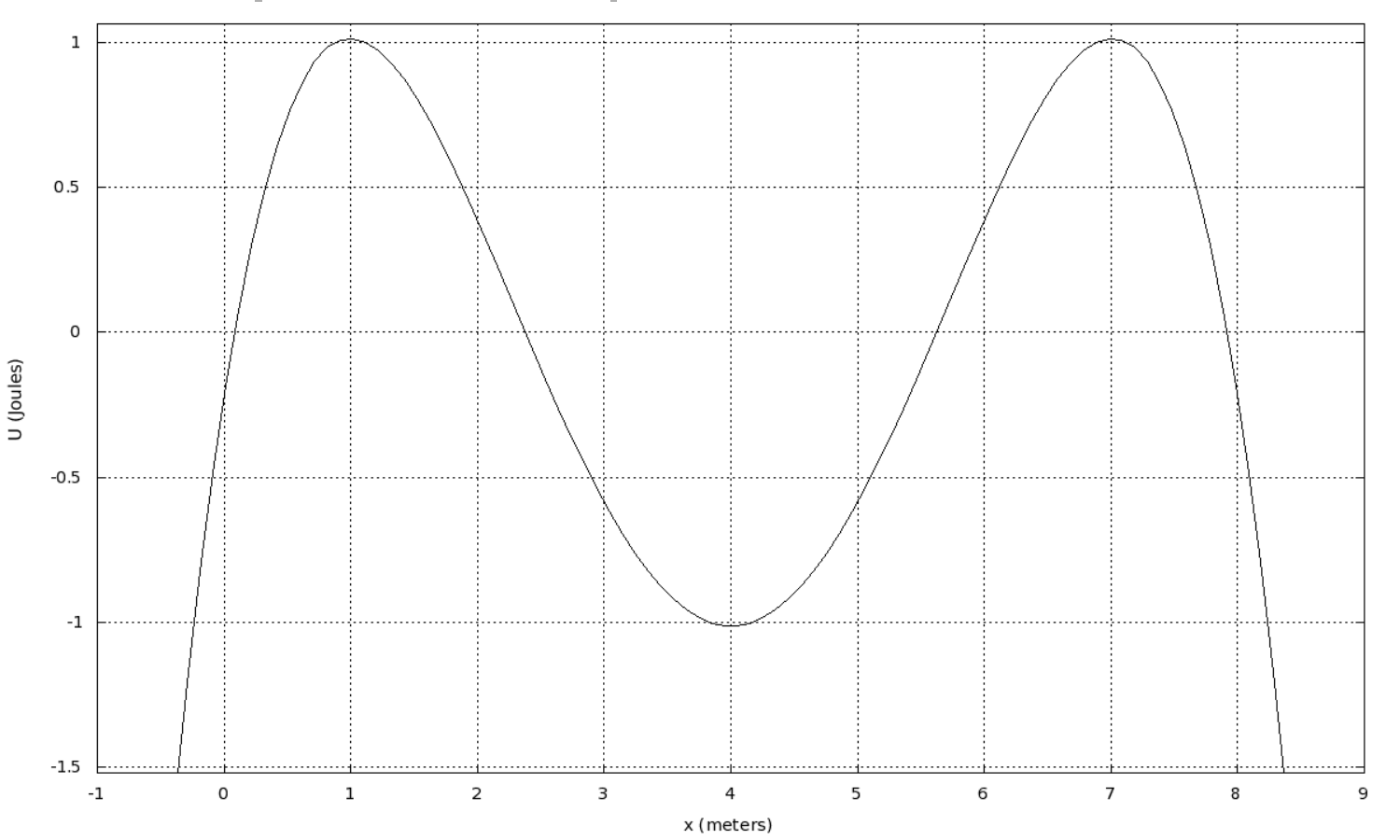Question
63 views

Shown below is a graph of potential energy as a function of position for a certain particle. Find the three equilibrium points, and decide whether each equilibrium is "stable" or "unstable." Let x1 be the equilibrium point with the smallest value of position. That means that x2 will have the next largest value, and then x3 will be the equilibrium point with the largest value of position. Don't forget units!

I found these questions:

1. x1 = 1.0 m
Unstable: Is this equilibrium point stable or unstable?

x2 = 4.0 m
Stable: Is this equilibrium point stable or unstable?

x3 = 7.0 m
Unstable: Is this equilibrium point stable or unstable?
2. Assume that the particle is released from rest at x = 6.0 m. The particle will start moving, but it will be bound to a certain region. What are the left and and right bounds (the turning points), xL and xR?
• xL = 2.0 m
• xR = 6.0 m

This is my question: Given the initial conditions, what is the maximum kinetic energy the particle can have?help_outlineImage Transcriptionclose1 0.5 -0.5 -1 -1.5 6 7 8 -1 1 2 3 5 x (meters) U (Joules) fullscreen
check_circle

star
star
star
star
star
1 Rating
Step 1

Given the particle is released from the point x=6 m.

...

### Want to see the full answer?

See Solution

#### Want to see this answer and more?

Solutions are written by subject experts who are available 24/7. Questions are typically answered within 1 hour.*

See Solution
*Response times may vary by subject and question.
Tagged in

### Kinematics